This dataset contains 8108 individuals and 16 variables, 1 quantitative variable is considered as illustrative.

### 1. Study of the outliers

The analysis of the graphs leads to detect outliers that strongly influence the results. First we will describe these outliers and then we will suppress them from the analysis. Looking at the graph, we can note that 3 particular individuals strongly contribute to the construction of the plane. The cumulative contribution of these individuals to the construction of the plane equals 82.2%.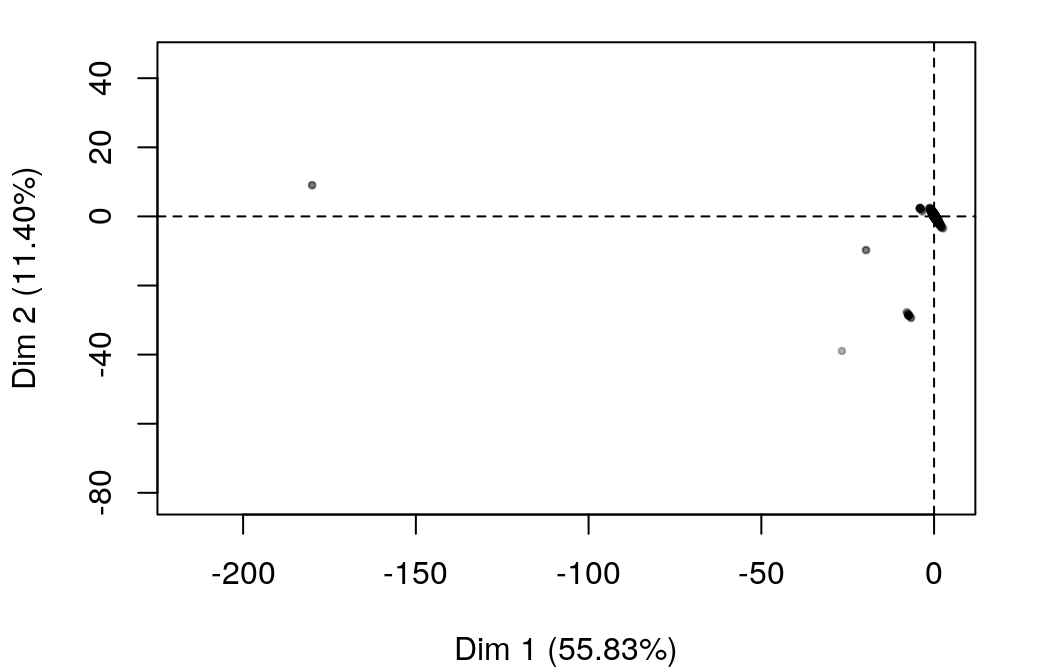Figure 1.1 - Individuals factor map (PCA) before correction. Highlighting of 3 outliers.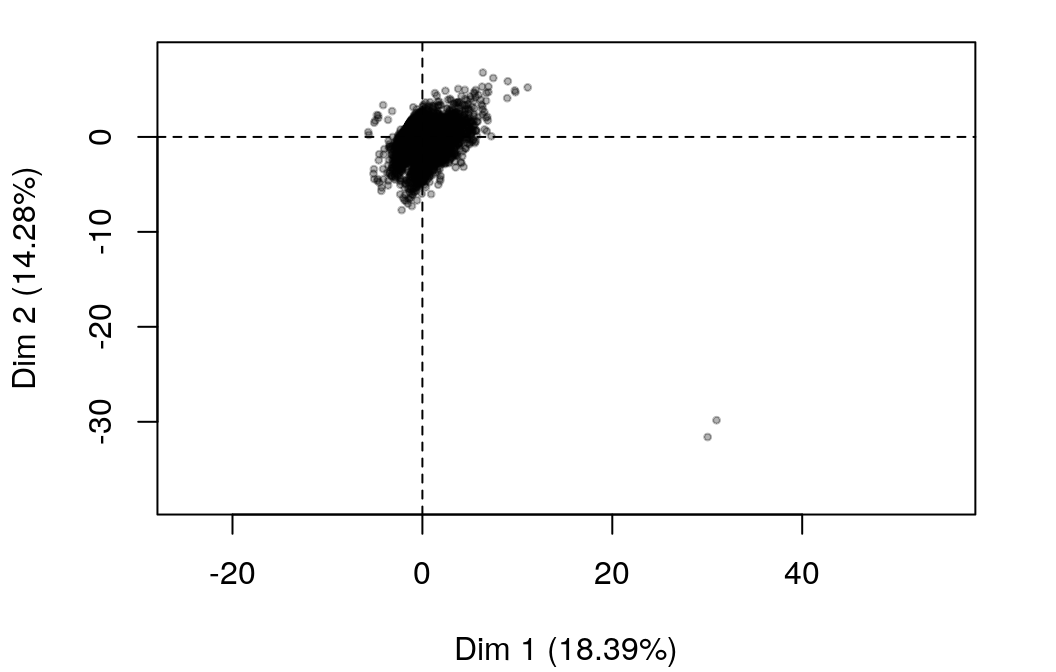Figure 1.2 - Individuals factor map (PCA) after correction. Highlighting of 3 outliers.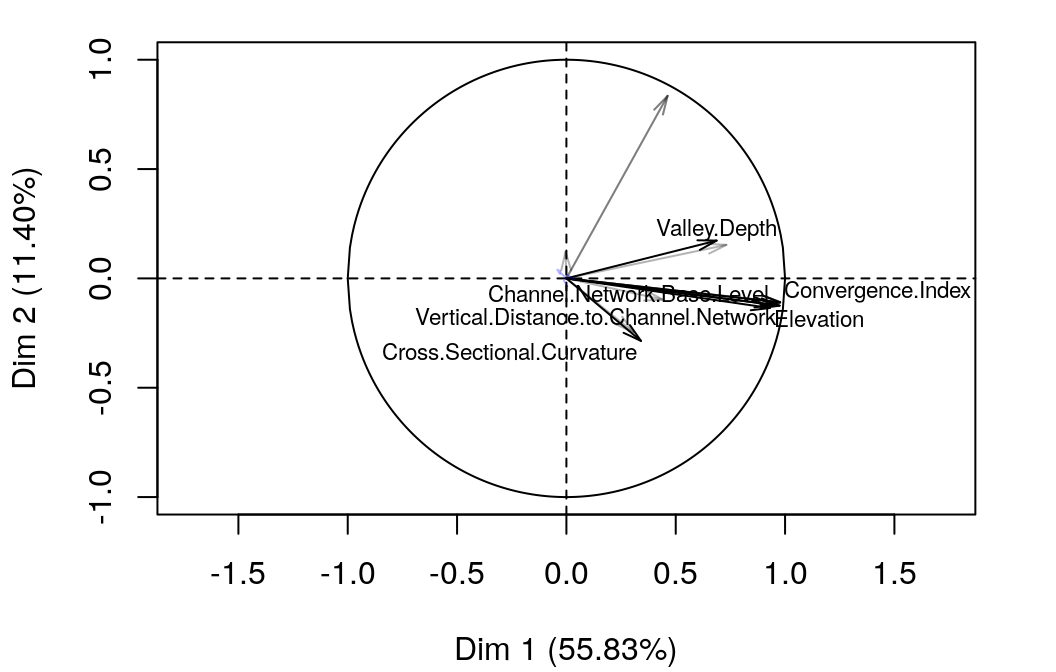Figure 1.3 - Variables factor map (PCA) before correction The labeled variables are those the best shown on the plane.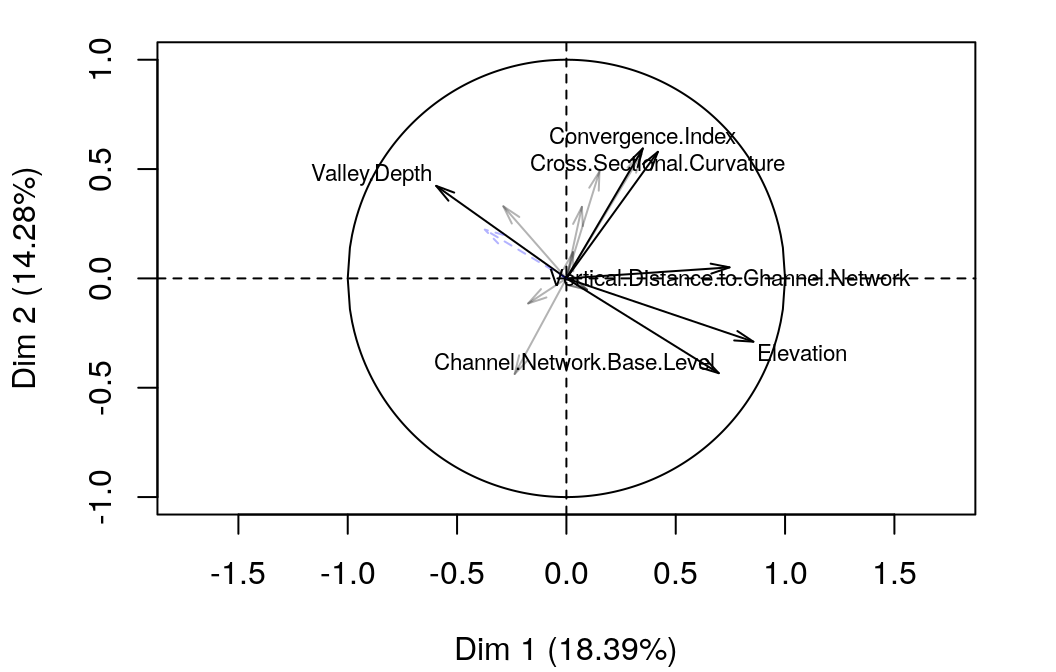Figure 1.3 - Variables factor map (PCA) after correction

The individual 5162 :

• takes very high values for the variable(s) :
• takes very low values for the variable(s) : Relative.Slope.Position, Valley.Depth, Topographic.Wetness.Index and LS.Factor (variables are sorted from the strongest).

The individual 5267 :

• takes very high values for the variable(s) :
• takes very low values for the variable(s) : Slope, Analytical.Hillshading, Catchment.Area, Convergence.Index, Vertical.Distance.to.Channel.Network, Channel.Network.Base.Level, Elevation, Relative.Slope.Position, Valley.Depth and Aspect (variables are sorted from the strongest).

The individual 6377 :

• takes very high values for the variable(s) :
• takes very low values for the variable(s) : Slope, Analytical.Hillshading, Catchment.Area, Convergence.Index, Vertical.Distance.to.Channel.Network, Channel.Network.Base.Level, Elevation, Relative.Slope.Position, Valley.Depth and Aspect (variables are sorted from the strongest).

These outliers are suppressed from the analysis and a second one is performed on the rest of the individuals.

### 2. Inertia distribution

The inertia of the first dimensions shows if there are strong relationships between variables and suggests the number of dimensions that should be studied.

The first two dimensions of PCA express 32.67% of the total dataset inertia ; that means that 32.67% of the individuals (or variables) cloud total variability is explained by the plane. This is an intermediate percentage and the first plane represents a part of the data variability. This value is greater than the reference value that equals 14.34%, the variability explained by this plane is thus significant (the reference value is the 0.95-quantile of the inertia percentages distribution obtained by simulating 547 data tables of equivalent size on the basis of a normal distribution).

From these observations, it may be interesting to consider the next dimensions which also express a high percentage of the total inertia.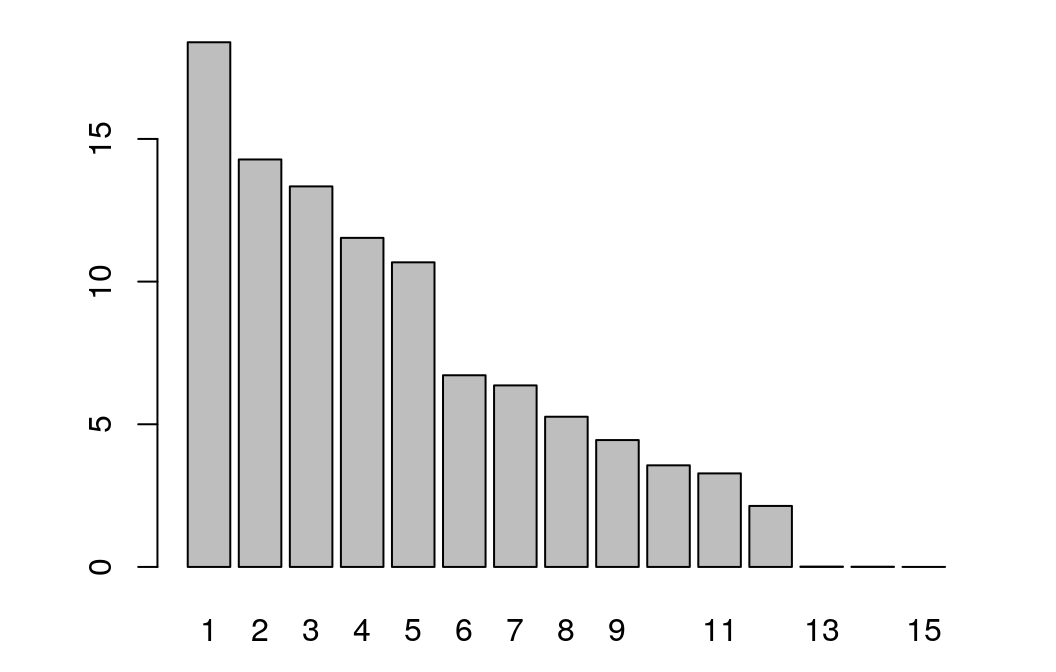Figure 2 - Decomposition of the total inertia on the components of the PCA

We can observe that the first 5 axis present an amount of inertia greater than those obtained by the 0.95-quantile of random distributions (68.22% against 35.11%). Thus, a wise decision would be to restrict the description to these only axis. However, we choosed to describe the first 8 axis.

### 3. Description of the plane 1:2Figure 3.1 - Individuals factor map (PCA) The labeled individuals are those with the higher contribution to the plane construction.